# Perimeter

The perimeter is the distance around the outside of the object.

To determine the perimeter, just add the lengths of the sides together.

Examples:

1.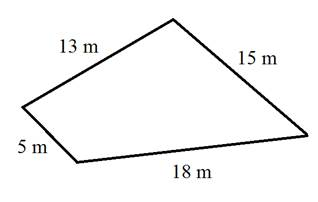Perimeter = 5 m + 13 m + 15 m + 18 m

Perimeter = 18 m + 15 m + 18 m

Perimeter = 33 m + 18 m

Perimeter = 51 m

2.This example shows a rectangle. The opposite sides are congruent.

We can just add all four sides:

P = 9 cm + 24 cm + 9 cm + 24 cm

P = 66 cm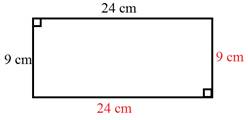Or we can use a perimeter formula for rectangles. The l stands for length and the w stands for width. It does not matter which side you call the length and which you call the width.

P = 2(l + w)

= 2(24 cm + 9 cm)

= 2 (33 cm)

= 66 cm

Or P = 2l + 2w

= 2(24 cm) + 2(9 cm)

= 48 cm + 18 cm

= 66 cm

3.)The dashes tell us that the two sides are congruent or equal.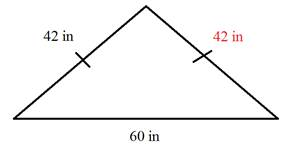Therefore, we can add up the three sides.

P = 42 in + 42 in + 60 in

P = 144 in

4.)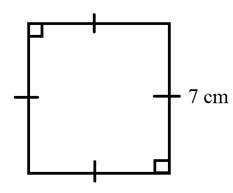Here we have a square. All 4 sides are the same.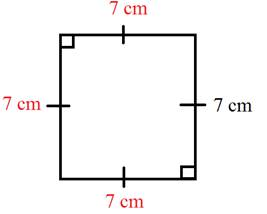We could just add all 4 sides.

P = 7 cm + 7 cm + 7 cm + 7 cm

= 28 cm

Or there is a formula for squares.

P = 4s (The s stands for side length.)

P = 4(7 cm)

P = 28 cm

5.) Kendra's back yard is in the shape of a rectangle. The length is 25 meters and the
width is 20meters. How much fencing would she need to wrap around the
entire back yard?

Start by drawing a picture!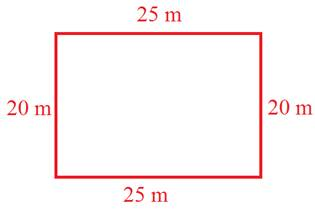Fencing would go around the outside of the shape. Therefore, we need the perimeter of the rectangle.

P = 2(l + w)

P = 2(25 m + 20 m)

P = 2(45 m)

P = 90 m

Kendra needs 90 meters of fencing to go around the entire yard.

Let's Review

To determine the perimeter, you need to add together the side lengths.

For some special shapes, there are formulas. However, if you do not know the formulas or forget the formulas, you can just add all the side lengths together.

 Related Links: Math Geometry Perimeter game for square and rectangle Perimeter of Parallelogram Perimeter of Rectangle Perimeter of Square Perimeter of Trapezoid Perimeter of the Triangle